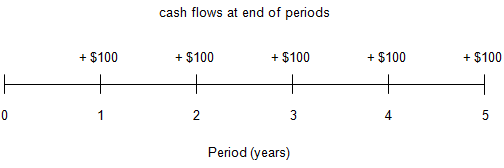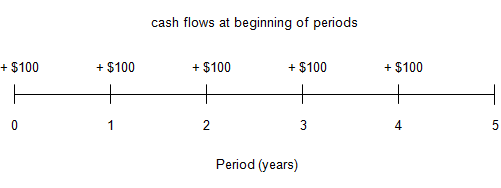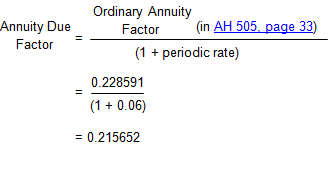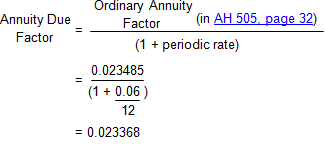Time Value of Money - Six Functions of a Dollar
Using Assessors Handbook Section 505 (Capitalization Formulas and Tables)
Appraisal Training: Self-Paced Online Learning Session

# Lesson 10: Annuities

This lesson discusses annuities in the context of the compound interest functions presented in Assessors’ Handbook Section 505 (AH 505), Capitalization Formulas and Tables. The lesson:

• Defines an annuity and two types of annuity,
• Explains how to convert an ordinary annuity factor into the corresponding annuity due factor, and
• Contains examples of converting annuity factors.

## Definition of an Annuity

An annuity is a series of equal cash flows, or payments, made at regular intervals (e.g., monthly or annually). The payments must be equal, and the interval between payments must be regular.

The following compound interest functions in AH 505 involve annuities:

• Future Worth of \$1 Per Period (FW\$1/P)
• Sinking Fund Factor (SFF)
• Present Worth of \$1 Per Period (PW\$1/P)
• Periodic Repayment (PR)

The two remaining compound interest functions -- the future worth of \$1 (FW\$1) and the present worth of \$1 (PW\$1) -- are not annuities because they apply to single payments rather than to a series of payments.

There are two types of annuities:

• Ordinary Annuity
• Annuity Due

## Ordinary Annuity

An ordinary annuity is an annuity in which the cash flows, or payments, occur at the end of the period.

An ordinary annuity of cash inflows of \$100 per year for 5 years can be represented like this:The cash flows occur at the end of years 1 through 5. And the first cash flow occurs at the end of year 1.

Most appraisal problems involve ordinary annuities; that is payments are assumed to occur at the end of the period. All of the formulas and factors in AH 505 pertain to ordinary annuities only.

## Annuity Due

An annuity due is an annuity in which the cash flows, or payments, occur at the beginning of the period. An annuity due is also called an annuity in arrears.

An annuity due of cash inflows of \$100 per year for 5 years can be represented like this:The cash flows occur at the beginning of years 1 through 5. And the first cash flow occurs at time 0 (now).

## Practical Applications Converting Annuity Factors

As noted, most appraisal problems assume that payments occur at the end of the period (ordinary annuity). But if payments occur at the beginning of the period (annuity due), an ordinary annuity factor in AH 505 can be converted to its corresponding annuity due factor with a relatively simple calculation.

Although financial calculators and spreadsheet software make it even easier to convert from an ordinary annuity to an annuity due, it is useful to understand how to "manually" convert the ordinary annuity factors in AH 505 to annuity due factors.

Conversion of ordinary annuity factor to annuity due factor for FW\$1/P or PW\$1/P:

To determine the Future Worth of \$1 Per Period (FW\$1/P) or Present Worth of \$1 Per Period (PW\$1/P) factor for an annuity due, refer to the corresponding factor in AH 505 for an ordinary annuity and multiply it by a factor of (1 + the periodic interest rate).

The periodic rate will differ depending on the compounding interval in the problem. For example, with annual compounding, the periodic rate would be the same as the annual rate; with monthly compounding the periodic rate would be the annual rate divided by 12.

Example 1: Conversion to annuity due factor for FW\$1/P
Calculate the FW\$1/P factor for 4 years at an annual interest rate of 6% with annual compounding, assuming payments occur at the beginning of each year.

Solution:
With annual compounding, the periodic rate equals the annual rate (6 percent, or 0.06).

• Annuity Due Factor = Ordinary Annuity Factor (in AH 505, page 33) × (1 + periodic rate)
• Annuity Due Factor = 4.374616 × (1 + 0.06)
• Annuity Due Factor = 4.637093

Example 2: Conversion to annuity due factor for PW\$1/P
Calculate the PW\$1/P factor for 4 years at an annual interest rate of 6% with monthly compounding, assuming payments occur at the beginning of each month.

Solution:
With monthly compounding, the periodic rate is 6% ÷ 12 = one-half of one percent per month, or 0.06 ÷ 12 = 0.005.

• Annuity Due Factor = Ordinary Annuity Factor (in AH 505, page 32) × (1 + periodic rate)
• Annuity Due Factor = 42.580318 × (1 + 0.005)
• Annuity Due Factor = 42.793220

Conversion of ordinary annuity factor to annuity due factor for SFF or PR:

To determine the Sinking Fund Factor (SFF) or Periodic Repayment (PR) Factor for an annuity due, refer to the corresponding factor in AH 505 for an ordinary annuity and divide it by a factor of (1+ the periodic interest rate). Be sure to divide, not multiply, when converting the SFF and PR factors. Note: the periodic rate will differ depending on the compounding interval in the problem.

Example 3: Conversion to annuity due for SFF
Calculate the SFF for 4 years at an annual interest rate of 6% with annual compounding, assuming payments occur at the beginning of each year.

Solution:Example 4: Conversion to annuity due for PR
Calculate the PR factor for 4 years at an annual interest rate of 6% with monthly compounding, assuming payments occur at the beginning of each month (monthly annuity due factor).

Solution: